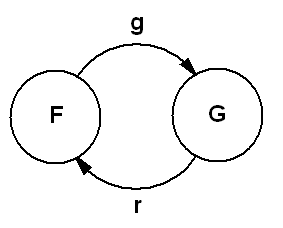Master of Science in Engineering University of Siena Automata and Queueing Systems Discrete Event Systems October 2016 - January 2017

## 1  News

• NEW! The results of the written test of March 1, 2017 are available in Section 4.3.
• See Section 4.1 for the learning assessment procedures.
• Students who passed the written test are requested to send an email to the Instructor to schedule a date for the oral test.
• Please read here the instructions to obtain and deliver a project.

## 2  About the instructor

### 2.1  Instructor

 Simone Paoletti, PhD Assistant Professor Building: San Niccolò Floor: 2 Room: 229 (red circle on the map) Email: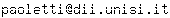Phone: 0577 23 4850 ext. 1020 Web: http://www3.diism.unisi.it/~paoletti/

### 2.2  Office hours

• Contact the Instructor via email.

## 3  About the course

### 3.1  Training objectives

• Discrete event systems are systems whose dynamic behaviour is driven by asynchronous occurrences of discrete events.
• Examples can be found in a variety of fields, such as control, computer science, automated manufacturing, and communication and transportation networks.
• The aim of this course is to introduce several modelling, simulation and analysis tools for discrete event systems.
• Lab tutorials with Matlab will be an important part of this course.

### 3.2  Required background

• Dynamical systems
• Probability

### 3.3  Organization

• The main course is DISCRETE EVENT SYSTEMS.
• CFU: 9
• Duration:  ∼ 72 hours
• Students: MSc CAE-R&A
• The course AUTOMATA AND QUEUEING SYSTEMS is a portion of the main course.
• CFU: 6
• Duration:  ∼ 48 hours (i.e. the first 48 hours of the main course)
• Students: MSc CAE-IS, LM GEST

### 3.4  Syllabus

 ALL ( ∼ 48 hours)
• Logical models of discrete event systems
• Timed models of discrete event systems
• Stochastic timed models of discrete event systems
• Simulation of discrete event systems
• Continuous-time Markov chains
• Queueing theory
• Markovian queueing networks
 CAE-R&A only ( ∼ 24 hours)
• Discrete-time Markov chains
• Control applications of discrete event systems

### 3.5  Didactic methods

• The course will be organized in lectures (50%) and tutorials, held both in class (25%) and in computer lab (25%).

### 3.6  Reference book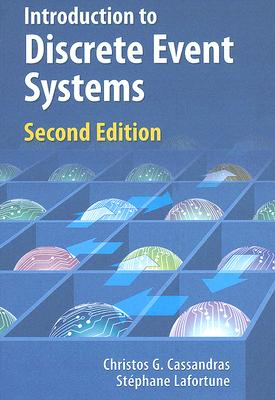[CL08] C.G. Cassandras, S. Lafortune, "Introduction to discrete event systems". 2nd ed., Springer, 2008.

## 4  Exams

### 4.1  Learning assessment procedures

 Final exam
• The final exam consists of both a written test and an oral test.
• The written test consists of exercises (typically three, to be solved in about 2.5 hours).
• Examples of past written tests (some with solutions) can be found in Section 4.2.
• Aid of Matlab is allowed (e.g. for matrix computations).
• The oral test is a broad-spectrum discussion on the topics of the course, including theory and exercises.
• Enabled only if the grade of the written test is ≥ 18 out of 30.
• To be given within the same session as the written test.
• In case of failure, the student must repeat also the written test.
• The language for the oral test can be either English or Italian
• The final grade is a weighted average of the grades of both tests.
 Midterm test, endterm test and project
• The written test of the final exam of AUTOMATA AND QUEUEING SYSTEMS can be replaced with:
• a midterm test (topics: logical, timed and stochastic timed models of DESs);
• a Matlab project (topics: simulation, continuous-time Markov chains, queueing systems).
• The written test of the final exam of DISCRETE EVENT SYSTEMS can be replaced with:
• a midterm test (topics: logical, timed and stochastic timed models of DESs);
• an endterm test (topics: discrete-time Markov chains);
• a Matlab project (topics: simulation, continuous-time Markov chains, queueing systems).
• Test(s) and project will receive a grade.
• Oral exam enabled only if the average grade is ≥ 18 and all grades ≥ 15 (out of 30).
• During the winter session of exams, one can improve the grade of either tests (not both).

### 4.2  Tests

 Past courses

## 6  About the lectures

### 6.1  Timetable

• The course ended on January 18, 2017.

### 6.2  Lecture schedule

 Date Topics Where to study   (B = book; LN = lecture notes) Additional material October 3, 2016 (2h) Presentation of the course October 4, 2016 (3h) Basics of system theory Concept of system Static vs dynamical systems Concept of state Continuous vs discrete state Concept of event Time-driven vs event-driven systems Systems vs (mathematical) models Definition of Discrete Event System (DES) [B] Chapter 1 (except §1.2.7, §1.2.8 and §1.3.5)       [LN] Chapter 1 October 5, 2016 (2h) Untimed models of DES: state automata (with outputs)    Graphical representation of state automata Example: queueing system [B] Section 2.2.2    [B] Example 2.11    [LN] Chapter 2 October 10, 2016 (2h) Exercises Exercises (with solutions)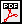October 11, 2016 (3h) Introduction to event timing Example: FIFO vs Round-Robin queueing Definition of clock structure Timed models of DES: timed automata [B] Section 5.1    [B] Section 5.2 (only §5.2.1)    [LN] Section 3.1 October 12, 2016 (2h) Basic examples of event timing dynamics Algorithm for event timing dynamics [B] Section 5.2 (only §5.2.2, §5.2.4 and §5.2.5) October 17, 2016 (2h) Example: FIFO vs Round-Robin queueing (revisited) October 18, 2016 (3h) Exercises Exercises (with solutions)October 19, 2016 (2h) Review of probability theory [B] Appendix I (except §I.7 and §I.8) Note di calcolo delle probabilità(in Italian) October 24, 2016 (2h) Uncertainty sources in models of DES Models of DES with uncertainty: stochastic timed automata [B] Sections 6.1, 6.3 and 6.4 October 25, 2016 (3h) Analysis of stochastic timed automata Example October 26, 2016 (2h) The exponential distribution: definition and properties November 7, 2016 (2h) Stochastic timed automata with Poisson clock structure Distribution of residual lifetimes Distribution of interevent times Distribution of events Distribution of states [B] Section 6.8 November 8, 2016 (3h) The Poisson counting process Stochastic timed automata with Poisson clock structure Distribution of state holding times Example [B] Sections 6.6 and 6.7 November 9, 2016 (2h) Exercise Exercise (with solutions)November 14, 2016 (2h) Exercises Exercises (with solutions)November 15, 2016 (3h) Exercises Exercises (with solutions)November 16, 2016 (2h) Exercises Exercises (with solutions)November 21, 2016 (2h) Midterm test (ALL) November 22, 2016 (3h) Basics of stochastic processes Markov property and Markov processes Continuous-time homogeneous Markov chains Chapman-Kolmogorov equations [B] Section 6.2    [B] Sections 7.1 and 7.3 (only §7.3.1 and §7.3.4) November 23, 2016 (2h) Continuous-time homogeneous Markov chains Transition rate matrix and its properties State holding times Transition probabilities Estimation of transition rates [B] Section 7.3 (only §7.3.5, §7.3.6 and §7.3.7) November 28, 2016 (2h) Continuous-time homogeneous Markov chains State probabilities Graphical representation Classification of states [B] Section 7.3 (only §7.3.8 and §7.3.9) November 29, 2016 (3h) Continuous-time homogeneous Markov chains Birth-death chains Steady state analysis Equivalences between classes of models Stochastic timed automata with Poisson clock structure    Continuous-time homogeneous Markov chains Example [B] Section 7.3 (only §7.3.10)       [B] Section 7.4 Exercises (with solutions)Matlab files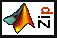November 30, 2016 (2h) Use of simulation for analysis of stochastic timed automata      Law of large numbers Estimation of state and event probabilities December 6, 2016 (3h) Queueing theory Specification of queueing models A/B/m/K notation Transient and steady state analysis Characterization of steady state Performance of queueing systems Little's law PASTA property [B] Sections from 8.1 to 8.5 December 7, 2016 (2h) Examples of Markovian queueing systems Exercises [B] Section 8.6 Exercises (with solutions)December 19, 2016 (2h) Simulation of stochastic timed automata (lab tutorial) December 21, 2016 (2h) Simulation of stochastic timed automata (lab tutorial) January 9, 2017 (2h) Discrete-time homogeneous Markov chains Chapman-Kolmogorov equations Transition probability matrix and its properties Graphical representation [B] Sections 7.1 and 7.2 (from §7.2.1 to §7.2.4) January 10, 2017 (3h) Discrete-time homogeneous Markov chains State probabilities State holding times Classification of states [B] Section 7.2 (from §7.2.5 to §7.2.8) January 16, 2017 (2h) Discrete-time homogeneous Markov chains Steady state analysis Applications to robotics [B] Section 7.2 (from §7.2.9 to §7.2.10) January 17, 2017 (3h) Exercises Exercises (with solutions)January 18, 2017 (2h) Exercises [B] Example 7.2 Exercises (with solutions)January 31, 2017 (1h) Endterm test (CAE-R&A only)

File translated from TEX by TTH, version 4.03.
On 25 May 2017, 13:09.